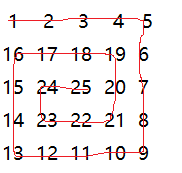# kanch.blog

posted at 2015-11-16 22:57:20 +0000

# C语言实现螺旋矩阵输出## 法一

（左侧为n=4，右侧为n=5）

``````//用来根据n是偶数还是奇数来决定最外层循环次数
if (n % 2 == 0) {
loops = n * n;
} else if (n % 2 != 0) {
loops = n * n - 1;
}
//now start to fill the arrary .....此处代码省略.....
//如果n为奇数，我们就单独设置最中间的值
if (n % 2 != 0) {
spi[(n - 1) / 2][(n - 1) / 2] = n * n;
}
``````

`````` //now start to fill the arrary
//rtop当前顶部行的位置，cright当前右侧列的位置，其它的我想我不用解释了
for (i = 1; i <= loops;) {
posp--; //这个变量针对底部行，左侧列的输出控制
//for the top-like raw
rtop++;
for (j = rtop; j < (xp + rtop); j++) {
spi[rtop][j] = i;
i++; //printf("%d ", spi[rtop][j]); }

//printf("n");
if (i > loops) {
break;
}

//for the right-like column
cright--;
for (j = rtop; j < (xp + rtop); j++) {
spi[j][cright] = i;
i++;
//printf("%d ", spi[j][cright]);
}

//printf("n");
if (i > loops) {
break;
}

//for the buttom-like raw
rbuttom--;
pb = posp;
for (j = xp; j > 0; j--) {
spi[rbuttom][pb] = i;
i++;
pb--;
//printf("%d ", spi[rbuttom][j]);
}

//printf("n");
if (i > loops) {
break;
}

//for the left-like raw
cleft++;
pb = posp;
for (j = xp; j > 0; j--) {
spi[pb][cleft] = i;
i++;
pb--;
//printf("%d ", spi[j][cleft]);
}

//printf("n");
if (i > loops) {
break;
}

//back-all processions
xp -= 2; //这个是记录每次输出多少个数字的
}
``````

<kanch@11/16/2015@CUIT>

`© kanch`zl AT kanchz DOT com

last updated on 2019-11-12 02:36:10 +0000